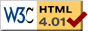### Journée-séminaire de combinatoire

#### (équipe CALIN du LIPN, université Paris-Nord, Villetaneuse)

Le 21 octobre 2014 à 14h00 en B107, Mathilde Bouvel nous parlera de : A general theory of Wilf-equivalence for Catalan structures

Résumé : It is a commonly observed phenomenon in enumerative combinatorics that several combinatorial classes share the same enumeration. For any two classes which seem to have the same enumeration sequence, a natural problem is to prove that it is indeed the case, ideally with a bijective proof that allows to map the structure of one class to that of the other. Such coincidences of enumeration are called Wilf-equivalences in the context of pattern-avoiding permutation classes (the definition of pattern-avoidance will be given during the talk). Wilf-equivalence has been a popular topic in the research on pattern-avoiding permutations, from its beginnings in the seventies until now. It is fair to say that most of the works done so far are specific to given pairs of equi-numerous classes, thus forming a sort of "case-by-case catalogue" of the known Wilf-equivalences. In this talk, we explore a different approach: we are interested in describing all Wilf-equivalences between permutations classes defined by the avoidance of two patterns: 231 and an additional pattern p (w.l.o.g., we can assume that p itself avoids 231). We will explain that this is one way of phrasing a seemingly more general (but actually equivalent) question: that of describing all Wilf-equivalences between classes of Catalan objects subject to one further avoidance restriction. Such classes are denoted Av(A), A being a Catalan object. Our results, to be presented in the talk, are the following. First, we define an equivalence relation ~ among Catalan objects. Our main result is that it refines Wilf-equivalence: if A ~ B, then Av(A) and Av(B) have the same enumeration. The proof is subdivided in several cases, and it is bijective in all cases but one. We further conjecture that the converse statement holds, i.e., that this relation ~ coincides with Wilf-equivalence. Then, we show how to enumerate the number of equivalence classes for ~, hereby providing an upper bound on the number of Wilf-equivalence classes. It is also interesting to study a special ~-equivalence class, which can be understood at a very fine level of details. Our results on this ~-class (called the "main" one) unify and generalize several results of the literature on Wilf-equivalence. Finally, we explain how our bijective cases in the proof of the main theorem can often be refined to provide comparison results between the enumerations of classes Av(A) and Av(B) when A and B are not equivalent for ~. A consequence is that the "main" ~-class corresponds to the largest possible enumeration sequence. This is joint work with Michael Albert (University of Otago), and a preprint is available at arXiv:1407.8261.

[arXiv]

 Dernière modification : Monday 24 January 2022Contact pour cette page : Cyril.Banderier at lipn.univ-paris13.fr